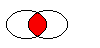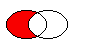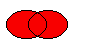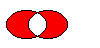# QRegion Class

The QRegion class specifies a clip region for a painter. More...

 Header: #include qmake: QT += gui

## Public Types

 enum RegionType { Rectangle, Ellipse } typedef const_iterator typedef const_reverse_iterator

## Public Functions

 QRegion(const QBitmap &bm) QRegion(QRegion &&other) QRegion(const QRegion &r) QRegion(const QPolygon &a, Qt::FillRule fillRule = Qt::OddEvenFill) QRegion(const QRect &r, QRegion::RegionType t = Rectangle) QRegion(int x, int y, int w, int h, QRegion::RegionType t = Rectangle) QRegion() QRegion & operator=(QRegion &&other) QRegion & operator=(const QRegion &r) QRegion::const_iterator begin() const QRect boundingRect() const QRegion::const_iterator cbegin() const QRegion::const_iterator cend() const bool contains(const QPoint &p) const bool contains(const QRect &r) const QRegion::const_reverse_iterator crbegin() const QRegion::const_reverse_iterator crend() const QRegion::const_iterator end() const QRegion intersected(const QRegion &r) const QRegion intersected(const QRect &rect) const bool intersects(const QRegion ®ion) const bool intersects(const QRect &rect) const bool isEmpty() const bool isNull() const QRegion::const_reverse_iterator rbegin() const int rectCount() const QRegion::const_reverse_iterator rend() const void setRects(const QRect *rects, int number) QRegion subtracted(const QRegion &r) const void swap(QRegion &other) void translate(int dx, int dy) void translate(const QPoint &point) QRegion translated(int dx, int dy) const QRegion translated(const QPoint &p) const QRegion united(const QRegion &r) const QRegion united(const QRect &rect) const QRegion xored(const QRegion &r) const QVariant operator QVariant() const bool operator!=(const QRegion &other) const const QRegion operator&(const QRegion &r) const const QRegion operator&(const QRect &r) const QRegion & operator&=(const QRegion &r) QRegion & operator&=(const QRect &r) const QRegion operator+(const QRegion &r) const const QRegion operator+(const QRect &r) const QRegion & operator+=(const QRegion &r) QRegion & operator+=(const QRect &rect) const QRegion operator-(const QRegion &r) const QRegion & operator-=(const QRegion &r) bool operator==(const QRegion &r) const const QRegion operator^(const QRegion &r) const QRegion & operator^=(const QRegion &r) const QRegion operator|(const QRegion &r) const QRegion & operator|=(const QRegion &r)
 QDataStream & operator<<(QDataStream &s, const QRegion &r) QDataStream & operator>>(QDataStream &s, QRegion &r)

## Detailed Description

QRegion is used with QPainter::setClipRegion() to limit the paint area to what needs to be painted. There is also a QWidget::repaint() function that takes a QRegion parameter. QRegion is the best tool for minimizing the amount of screen area to be updated by a repaint.

This class is not suitable for constructing shapes for rendering, especially as outlines. Use QPainterPath to create paths and shapes for use with QPainter.

QRegion is an implicitly shared class.

### Creating and Using Regions

A region can be created from a rectangle, an ellipse, a polygon or a bitmap. Complex regions may be created by combining simple regions using united(), intersected(), subtracted(), or xored() (exclusive or). You can move a region using translate().

You can test whether a region isEmpty() or if it contains() a QPoint or QRect. The bounding rectangle can be found with boundingRect().

Iteration over the region (with begin(), end(), or C++11 ranged-for loops) gives a decomposition of the region into rectangles.

Example of using complex regions:

```void MyWidget::paintEvent(QPaintEvent *)
{
QRegion r1(QRect(100, 100, 200, 80),    // r1: elliptic region
QRegion::Ellipse);
QRegion r2(QRect(100, 120, 90, 30));    // r2: rectangular region
QRegion r3 = r1.intersected(r2);        // r3: intersection

QPainter painter(this);
painter.setClipRegion(r3);
...                                     // paint clipped graphics
}```

## Member Type Documentation

### enum QRegion::RegionType

Specifies the shape of the region to be created.

ConstantValueDescription
`QRegion::Rectangle``0`the region covers the entire rectangle.
`QRegion::Ellipse``1`the region is an ellipse inside the rectangle.

### typedef QRegion::const_iterator

An iterator over the non-overlapping rectangles that make up the region.

The union of all the rectangles is equal to the original region.

QRegion does not offer mutable iterators.

This typedef was introduced in Qt 5.8.

### typedef QRegion::const_reverse_iterator

A reverse iterator over the non-overlapping rectangles that make up the region.

The union of all the rectangles is equal to the original region.

QRegion does not offer mutable iterators.

This typedef was introduced in Qt 5.8.

## Member Function Documentation

### QRegion::QRegion(const QBitmap &bm)

Constructs a region from the bitmap bm.

The resulting region consists of the pixels in bitmap bm that are Qt::color1, as if each pixel was a 1 by 1 rectangle.

This constructor may create complex regions that will slow down painting when used. Note that drawing masked pixmaps can be done much faster using QPixmap::setMask().

### QRegion::QRegion(QRegion &&other)

Move-constructs a new region from region other. After the call, other is null.

This function was introduced in Qt 5.7.

### QRegion::QRegion(const QRegion &r)

Constructs a new region which is equal to region r.

### QRegion::QRegion(const QPolygon &a, Qt::FillRulefillRule = Qt::OddEvenFill)

Constructs a polygon region from the point array a with the fill rule specified by fillRule.

If fillRule is Qt::WindingFill, the polygon region is defined using the winding algorithm; if it is Qt::OddEvenFill, the odd-even fill algorithm is used.

Warning: This constructor can be used to create complex regions that will slow down painting when used.

### QRegion::QRegion(const QRect &r, QRegion::RegionTypet = Rectangle)

Create a region based on the rectangle r with region type t.

If the rectangle is invalid a null region will be created.

### QRegion::QRegion(intx, inty, intw, inth, QRegion::RegionTypet = Rectangle)

Constructs a rectangular or elliptic region.

If t is `Rectangle`, the region is the filled rectangle (x, y, w, h). If t is `Ellipse`, the region is the filled ellipse with center at (x + w / 2, y + h / 2) and size (w ,h).

### QRegion::QRegion()

Constructs an empty region.

### QRegion &QRegion::operator=(QRegion &&other)

Move-assigns other to this QRegion instance.

This function was introduced in Qt 5.2.

### QRegion &QRegion::operator=(const QRegion &r)

Assigns r to this region and returns a reference to the region.

### QRegion::const_iterator QRegion::begin() const

Returns a const_iterator pointing to the beginning of the range of non-overlapping rectangles that make up the region.

The union of all the rectangles is equal to the original region.

This function was introduced in Qt 5.8.

### QRect QRegion::boundingRect() const

Returns the bounding rectangle of this region. An empty region gives a rectangle that is QRect::isNull().

### QRegion::const_iterator QRegion::cbegin() const

Same as begin().

This function was introduced in Qt 5.8.

### QRegion::const_iterator QRegion::cend() const

Same as end().

This function was introduced in Qt 5.8.

### bool QRegion::contains(const QPoint &p) const

Returns `true` if the region contains the point p; otherwise returns `false`.

### bool QRegion::contains(const QRect &r) const

Returns `true` if the region overlaps the rectangle r; otherwise returns `false`.

### QRegion::const_reverse_iterator QRegion::crbegin() const

Same as rbegin().

This function was introduced in Qt 5.8.

### QRegion::const_reverse_iterator QRegion::crend() const

Same as rend().

This function was introduced in Qt 5.8.

### QRegion::const_iterator QRegion::end() const

Returns a const_iterator pointing to one past the end of non-overlapping rectangles that make up the region.

The union of all the rectangles is equal to the original region.

This function was introduced in Qt 5.8.

### QRegion QRegion::intersected(const QRegion &r) const

Returns a region which is the intersection of this region and r.The figure shows the intersection of two elliptical regions.

This function was introduced in Qt 4.2.

### QRegion QRegion::intersected(const QRect &rect) const

Returns a region which is the intersection of this region and the given rect.

This function was introduced in Qt 4.4.

### bool QRegion::intersects(const QRegion &region) const

Returns `true` if this region intersects with region, otherwise returns `false`.

This function was introduced in Qt 4.2.

### bool QRegion::intersects(const QRect &rect) const

Returns `true` if this region intersects with rect, otherwise returns `false`.

This function was introduced in Qt 4.2.

### bool QRegion::isEmpty() const

Returns `true` if the region is empty; otherwise returns `false`. An empty region is a region that contains no points.

Example:

```QRegion r1(10, 10, 20, 20);
r1.isEmpty();               // false

QRegion r3;
r3.isEmpty();               // true

QRegion r2(40, 40, 20, 20);
r3 = r1.intersected(r2);    // r3: intersection of r1 and r2
r3.isEmpty();               // true

r3 = r1.united(r2);         // r3: union of r1 and r2
r3.isEmpty();               // false```

### bool QRegion::isNull() const

Returns `true` if the region is empty; otherwise returns `false`. An empty region is a region that contains no points. This function is the same as isEmpty

This function was introduced in Qt 5.0.

### QRegion::const_reverse_iterator QRegion::rbegin() const

Returns a const_reverse_iterator pointing to the beginning of the range of non-overlapping rectangles that make up the region.

The union of all the rectangles is equal to the original region.

This function was introduced in Qt 5.8.

### int QRegion::rectCount() const

Returns the number of rectangles that this region is composed of. Same as `end() - begin()`.

This function was introduced in Qt 4.6.

### QRegion::const_reverse_iterator QRegion::rend() const

Returns a const_reverse_iterator pointing to one past the end of the range of non-overlapping rectangles that make up the region.

The union of all the rectangles is equal to the original region.

This function was introduced in Qt 5.8.

### void QRegion::setRects(const QRect *rects, intnumber)

Sets the region using the array of rectangles specified by rects and number. The rectangles must be optimally Y-X sorted and follow these restrictions:

• The rectangles must not intersect.
• All rectangles with a given top coordinate must have the same height.
• No two rectangles may abut horizontally (they should be combined into a single wider rectangle in that case).
• The rectangles must be sorted in ascending order, with Y as the major sort key and X as the minor sort key.

### QRegion QRegion::subtracted(const QRegion &r) const

Returns a region which is r subtracted from this region.The figure shows the result when the ellipse on the right is subtracted from the ellipse on the left (`left - right`).

This function was introduced in Qt 4.2.

### void QRegion::swap(QRegion &other)

Swaps region other with this region. This operation is very fast and never fails.

This function was introduced in Qt 4.8.

### void QRegion::translate(intdx, intdy)

Translates (moves) the region dx along the X axis and dy along the Y axis.

### void QRegion::translate(const QPoint &point)

Translates the region point.x() along the x axis and point.y() along the y axis, relative to the current position. Positive values move the region to the right and down.

Translates to the given point.

### QRegion QRegion::translated(intdx, intdy) const

Returns a copy of the region that is translated dx along the x axis and dy along the y axis, relative to the current position. Positive values move the region to the right and down.

This function was introduced in Qt 4.1.

### QRegion QRegion::translated(const QPoint &p) const

Returns a copy of the regtion that is translated p.x() along the x axis and p.y() along the y axis, relative to the current position. Positive values move the rectangle to the right and down.

This function was introduced in Qt 4.1.

### QRegion QRegion::united(const QRegion &r) const

Returns a region which is the union of this region and r.The figure shows the union of two elliptical regions.

This function was introduced in Qt 4.2.

### QRegion QRegion::united(const QRect &rect) const

Returns a region which is the union of this region and the given rect.

This function was introduced in Qt 4.4.

### QRegion QRegion::xored(const QRegion &r) const

Returns a region which is the exclusive or (XOR) of this region and r.The figure shows the exclusive or of two elliptical regions.

This function was introduced in Qt 4.2.

### QVariant QRegion::operator QVariant() const

Returns the region as a QVariant

### bool QRegion::operator!=(const QRegion &other) const

Returns `true` if this region is different from the other region; otherwise returns `false`.

### const QRegion QRegion::operator&(const QRegion &r) const

Applies the intersected() function to this region and r. `r1&r2` is equivalent to `r1.intersected(r2)`.

### const QRegion QRegion::operator&(const QRect &r) const

This function was introduced in Qt 4.4.

### QRegion &QRegion::operator&=(const QRegion &r)

Applies the intersected() function to this region and r and assigns the result to this region. `r1&=r2` is equivalent to `r1` = r1.intersected(r2).

### QRegion &QRegion::operator&=(const QRect &r)

This function was introduced in Qt 4.4.

### const QRegion QRegion::operator+(const QRegion &r) const

Applies the united() function to this region and r. `r1+r2` is equivalent to `r1.united(r2)`.

### const QRegion QRegion::operator+(const QRect &r) const

This function was introduced in Qt 4.4.

### QRegion &QRegion::operator+=(const QRegion &r)

Applies the united() function to this region and r and assigns the result to this region. `r1+=r2` is equivalent to `r1 = r1.united(r2)`.

### QRegion &QRegion::operator+=(const QRect &rect)

Returns a region that is the union of this region with the specified rect.

### const QRegion QRegion::operator-(const QRegion &r) const

Applies the subtracted() function to this region and r. `r1-r2` is equivalent to `r1.subtracted(r2)`.

### QRegion &QRegion::operator-=(const QRegion &r)

Applies the subtracted() function to this region and r and assigns the result to this region. `r1-=r2` is equivalent to `r1 = r1.subtracted(r2)`.

### bool QRegion::operator==(const QRegion &r) const

Returns `true` if the region is equal to r; otherwise returns false.

### const QRegion QRegion::operator^(const QRegion &r) const

Applies the xored() function to this region and r. `r1^r2` is equivalent to `r1.xored(r2)`.

### QRegion &QRegion::operator^=(const QRegion &r)

Applies the xored() function to this region and r and assigns the result to this region. `r1^=r2` is equivalent to `r1 = r1.xored(r2)`.

### const QRegion QRegion::operator|(const QRegion &r) const

Applies the united() function to this region and r. `r1|r2` is equivalent to `r1.united(r2)`.

### QRegion &QRegion::operator|=(const QRegion &r)

Applies the united() function to this region and r and assigns the result to this region. `r1|=r2` is equivalent to `r1 = r1.united(r2)`.

## Related Non-Members

### QDataStream &operator<<(QDataStream &s, const QRegion &r)

Writes the region r to the stream s and returns a reference to the stream.